Next: Particular Implementation of LSJIMP Up: The LSJIMP Inverse problem Previous: Combined LSJIMP Data and

## LSJIMP Nonlinear Iterations

Proponents of so-called full-waveform inversion'' Tarantola (1984) seek to solve an ambitious nonlinear inverse problem. In the context of reflection seismology, full-waveform techniques seek to invert a relation of the following qualitative form:

 recorded data =Most generally, the operatoris elastic two-way wave equation modeling, although making the acoustic assumption simplifies matters. In both cases, the modeling operator is non-linearly dependent on the earth model to be estimated. For many reasons (including, but not limited to: computational expense, model non-uniqueness/nullspace, and sensitivity to starting model), full-waveform techniques are rarely applied successfully in today's conventional seismic processing environment. The LSJIMP method can be abstracted in a similar qualitative fashion, using the notation of the previous sections: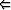velocity, reflection coefficient, crosstalk model =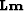Quantities like imaging velocity, the measured reflection coefficient of the multiple generators, and the crosstalk model are assumed to be fixed. Some LSJIMP implementations might depend only implicitly on velocity or reflection coefficient. For the sake of argument, however, let us assume that the LSJIMP operator,, is a non-linear function of these parameters, which the basic LSJIMP inversion makes no attempt to optimize. A multiple-free estimate of the primaries obviously enhances our ability to estimate imaging velocity, regardless of the method, and also permits us to model crosstalk noise from primaries that are below the onset of the seabed pure multiple. Thus the simplest nonlinear iteration of the LSJIMP method would proceed as follows, where the superscript k denotes that an operator or model vector is attached to thenonlinear iteration:iterate {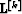velocity, reflection coefficients, crosstalk model =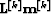updated velocity, crosstalk modelNonlinear updating of the reflection coefficients of the multiple generators is in general a more difficult, and potentially more valuable, problem. If a multiple generator's pure multiple is obscured by other events, the reflection coefficient estimation scheme outlined in sectionmay produce inaccurate estimates, which the spatial regularization may not account for. For example, in the 2-D field data example shown in Chapter, the pure multiple for one multiple generator happens to be overlapped over almost the entire line by a prominent pegleg from another multiple generator.

I propose a nonlinear reflection coefficient updating scheme which obtains perturbations by fitting unmodeled events in the LSJIMP data residual,. If the initial reflection coefficient is perfect, then after convergence,will contain only uncorrelated noise. If it is imperfect, then we also expect to see correlated events left over in the residual. Because LSJIMP separates each multiple mode indpendently in the model space (), we can simply apply the forward model for that mode () to obtain an estimate of the particular multiple in data space,.

The main idea of my updating scheme is to compute a scalar update to the reflection coefficient of themultiple generator,, such that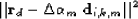(13)
is minimized. Sincemay contain correlated events from primaries and other multiple modes, I suggest usingto compute a residual weight which is large whereis energetic, small where it is not. Using the absolute value ofis one possibility:(14)
In the implementation of minimization () used in this thesis,is computed independently at each midpoint location, y. The updated reflection coefficient,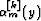, is related simply toand the previous reflection coefficient:(15)
where the superscripts again represent nonlinear iteration index.Next: Particular Implementation of LSJIMP Up: The LSJIMP Inverse problem Previous: Combined LSJIMP Data and
Stanford Exploration Project
5/30/2004